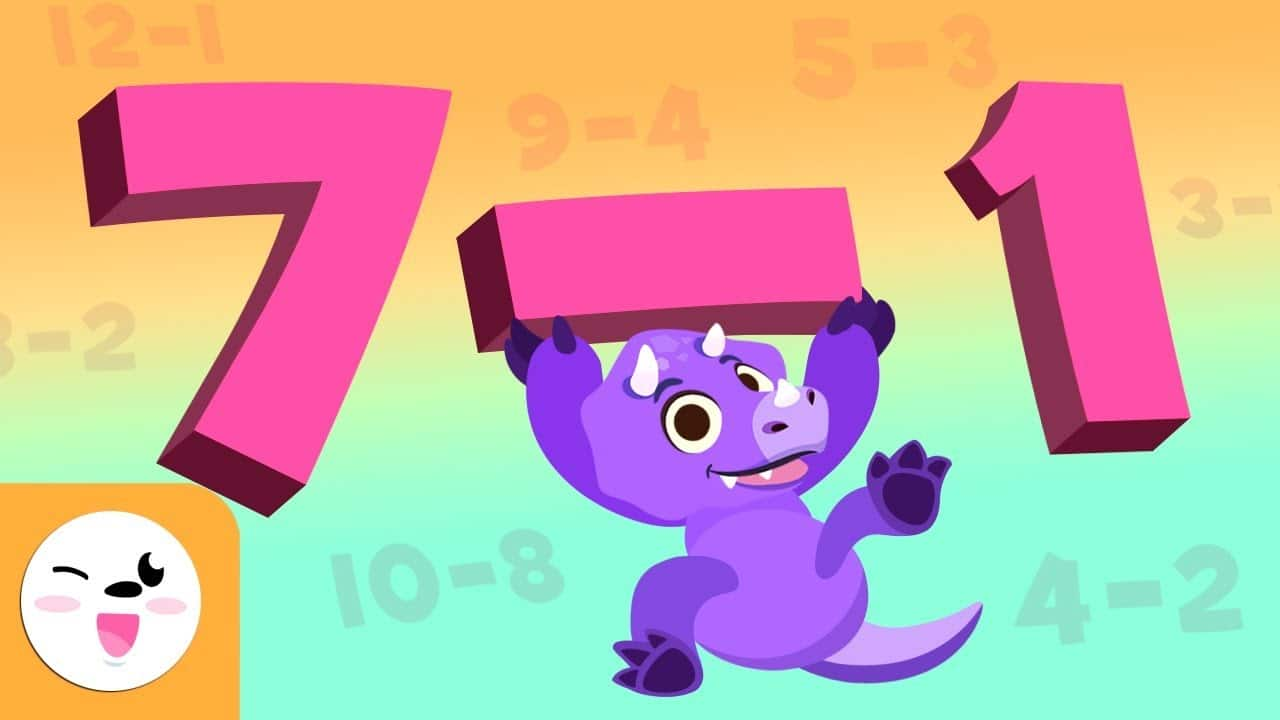Generic selectors
Exact matches only
Search in title
Search in content
Post Type Selectors
post
page# How to teach addition and subtraction to childrenAs we have explained in our previous post, teaching math to children can be a challenge, but with exercises and resources adapted to their needs we can make math more accessible for students.

In today’s blog post, we offer more tips, so that learning can be easy and fun. In addition, we have included a series of videos from our YouTube channel with practical exercises that can be used to teach addition and subtraction.

How to teach math to elementary school children

When it’s time to teach math to the little ones, we can face some problems such as:

• Lack of motivation. One of the most recurrent problems is the lack of motivation when doing math. Some students may feel like math is boring or difficult, which can lead to a lack of interest in learning.
• Difficulty in comprehension. The abstract nature of many math concepts can be very complicated for children. Comprehending these abstract terms can be an obstacle.
• Excessively theoretical focus. Sometimes, the focus of teaching math is centered heavily on the theory and not the practical application. Students may lose interest if they do not see the relevance of what they are learning applied to their everyday life.
• Lack of adequate resources. Some schools lack the educational and technological resources needed to effectively teach math, which limits the possibilities of interactive learning.

Tips to teach addition and subtraction to children

In order to address the previous problems, it is essential to promote the teaching of math in an accessible, relevant, and attractive way for students. We can achieve this through the incorporation of educational platforms, interactive games, application to real world scenarios during lessons…

Here we have several tips that can help you teach two basic mathematical operations to children: addition and subtraction.

1. Visualization through drawings. Utilize simple drawings and graphics to represent addition and subtraction problems. For example, draw apples to represent the amount of fruit in a box then add or subtract apples in order to resolve problems.
2. Mathematical tales. Look for tales and stories that include addition and subtraction.
3. Tables and graphics. Use simple tables and graphics to help children organize the information from addition and subtraction problems. This will help them visualize and resolve the problems in a more effective manner.
4. Incorporate the use of technology. Educational applications and online games can be useful to practice addition and subtraction in an interactive way. In addition, these tools can provide feedback instantly, which helps children correct errors and strengthen their skills.

A simple way to explain addition to children is by the following: addition is a mathematical operation that is used to join or combine two or more numbers in order to obtain a total number. When you add, you are combining quantities together. For example, if you add 2 and 3, you get 5, because you have combined those two numbers.

In this video, children will discover how easy it is to add thanks to Dino. You can also find various exercise examples with their corresponding answers.

Subtraction

Similarly to the previous case, a simple way to explain subtraction can be: subtraction is a mathematical operation that is used to take away one quantity from another. It is like taking things away from a group. For example, if you have 8 toys and you take away 4, you have 4 toys left.

In this video, the dinosaurs from Smile and Learn will teach children how to subtract using various examples like the previous ones.

Interactive math problems for children

Utilizing educational applications and online games specifically designed for teachers helps reinforce mathematical concepts like addition and subtraction while children have fun resolving these mathematical challenges. In addition, they’re fun! Here are two examples:

At Smile and Learn, we have a wide array of educational resources that will help children learn mathematical concepts such as addition and subtraction. In addition, in our app you can find more than 12,000 activities that cover curriculum content, develop key competencies, and much more!

If you still have not tried our educational platform, you can do so for free by filling out the following form.

## More entries

• #### Building confidence through reinforcing bilingualism

7 de November de 2023
• #### Tips to help children work on pragmatics

25 de October de 2023
• #### CONTEST!

4 de October de 2023
• #### How to teach addition and subtraction to children

3 de October de 2023